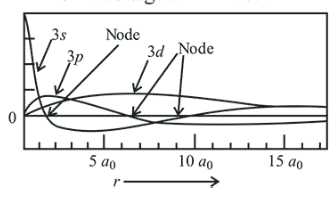# Which of the following combination of statements is trueQuestion:

Which of the following combination of statements is true regarding the interpretation of the atomic orbitals?

(a) An electron in an orbital of high angular momentum stays away from the nucleus than an electron in the orbital of lower angular momentum.

(b) For a given value of the principal quantum number, the size of the orbit is inversely proportional to the azimuthal quantum number.

(c) According to wave mechanics, the ground state angular momentum is equal to $\frac{\mathrm{h}}{2 \pi}$

(d) The plot of $\psi$ vs $r$ for various azimuthal quantum numbers, shows peak shifting towards higher $r$ value.

1. (a), (d)

2. $(\mathrm{a}),(\mathrm{b})$

3. (a), (c)

4. (b), (c)

Correct Option:

Solution:

(a) Angular momentum $(L)=\frac{n \mathrm{~h}}{2 \pi}$ Therefore, as $n$ increases, $L$ also increases.

(b) $\quad r \propto \frac{n^{2}}{\mathrm{Z}}$

(c) For $n=1, L=\frac{\mathrm{h}}{2 \pi}$

(d) As $l$ increases, the peak of $\psi$ vs $r$ shifts towards higher ' $r$ ' value.